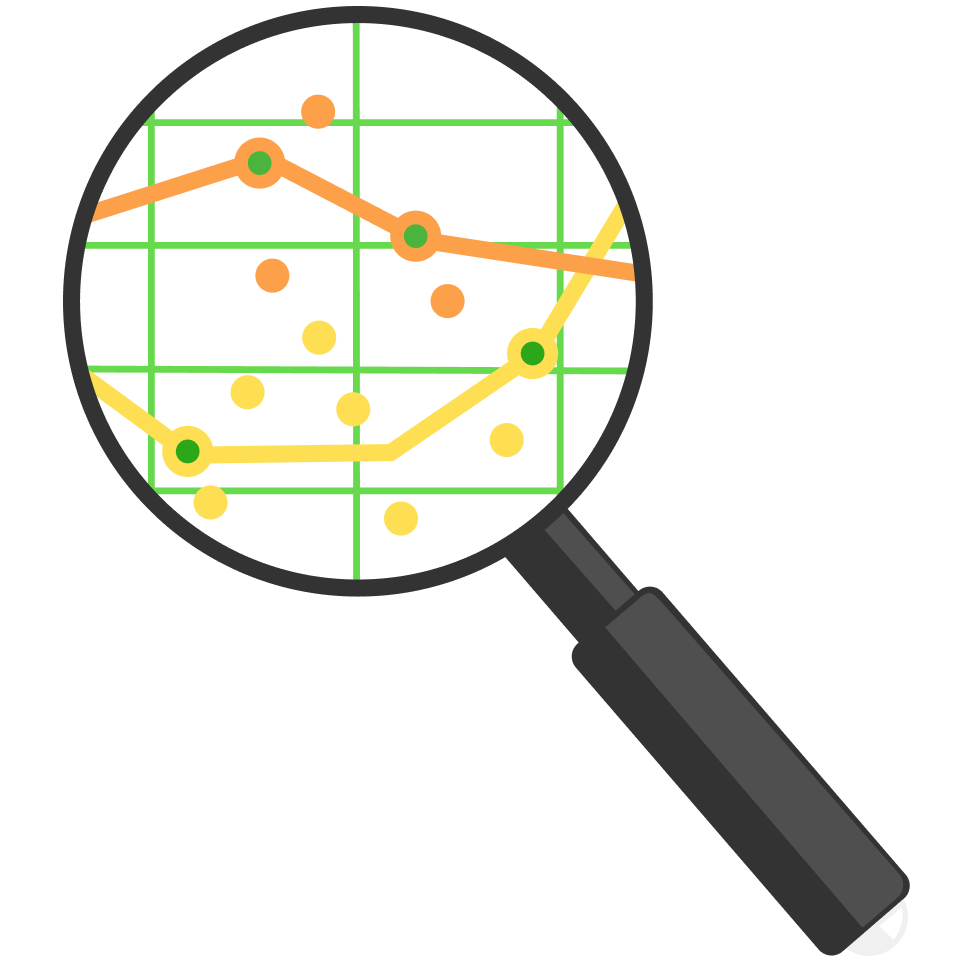# Piercing Lies With Statistics

The questions that follow let you experiment with real data, using Python code. You don't need to know anything about writing computer code!

In the code below, you can edit the first line to count the number of times particular digits occur in a string of numbers. Try it out before you go on to the first question.

digit_string = '12345678901234567890'

for i in range(10):
print('Occurrences of digit', str(i), '->', digit_string.count(str(i)))
Python 3
You need to be connected to run code

# Piercing Lies With Statistics

Suppose you ask someone to fill a page using random digits between 0 and 9 with no external tools like dice allowed. In a general sense, which chart is the data most likely to resemble?

Note that this is a psychology question! As mentioned at the end of the last quiz, we'll be considering some questions that aren't "pure math", because statistics always needs to account for context. It's recommended you use the code below the graphs to experiment; try typing a very long string of digits at random and let the program count how many times each digit occurred.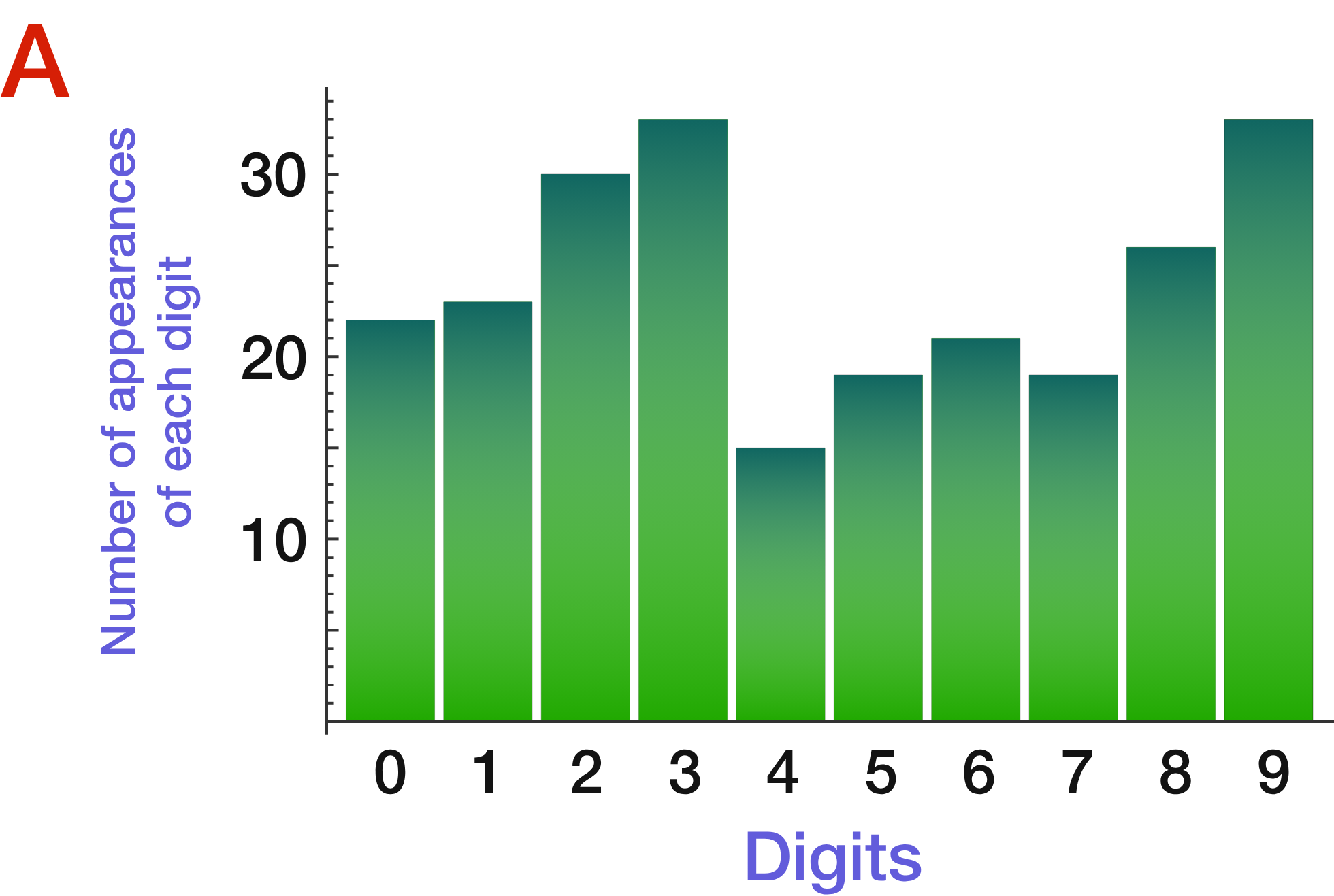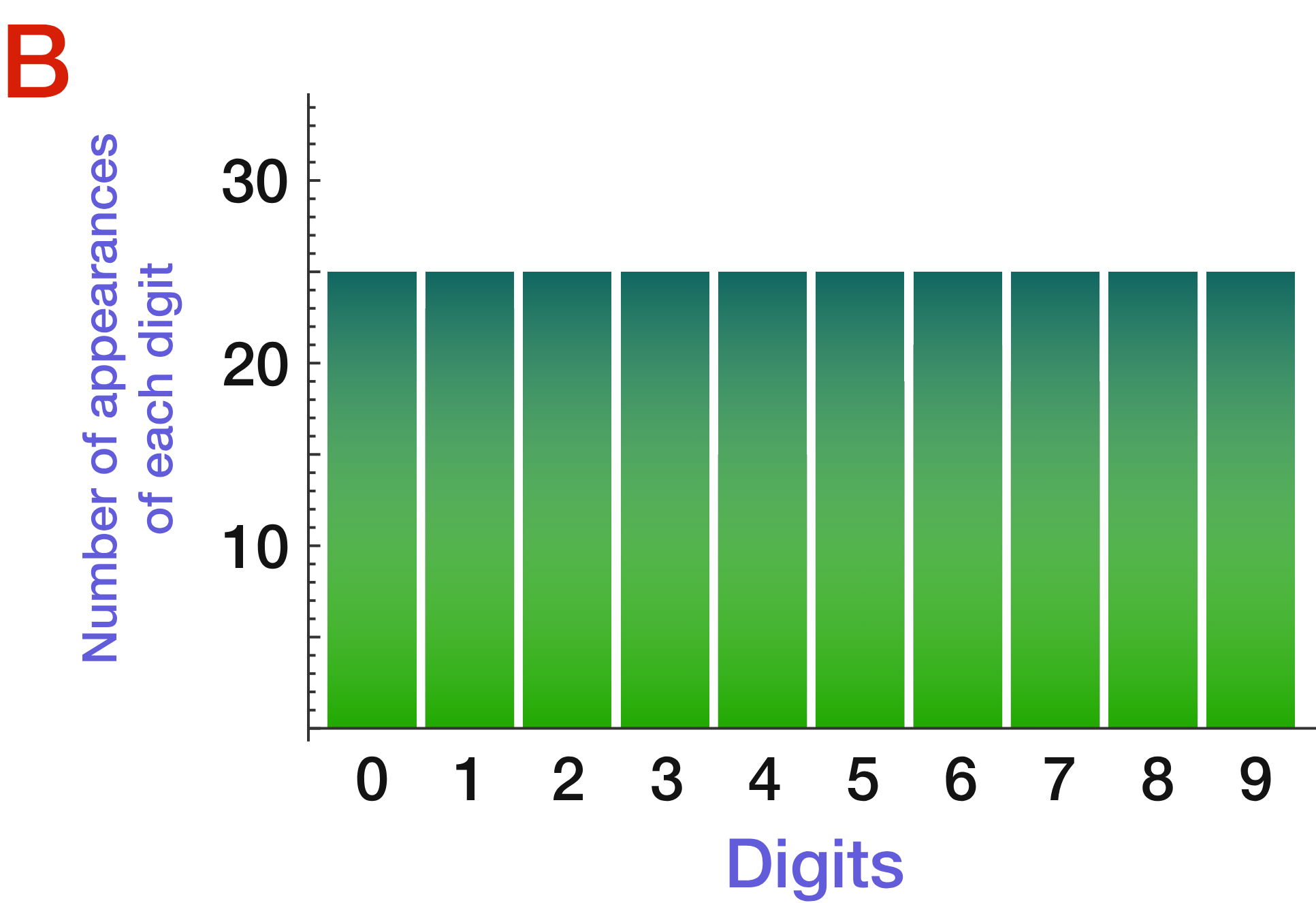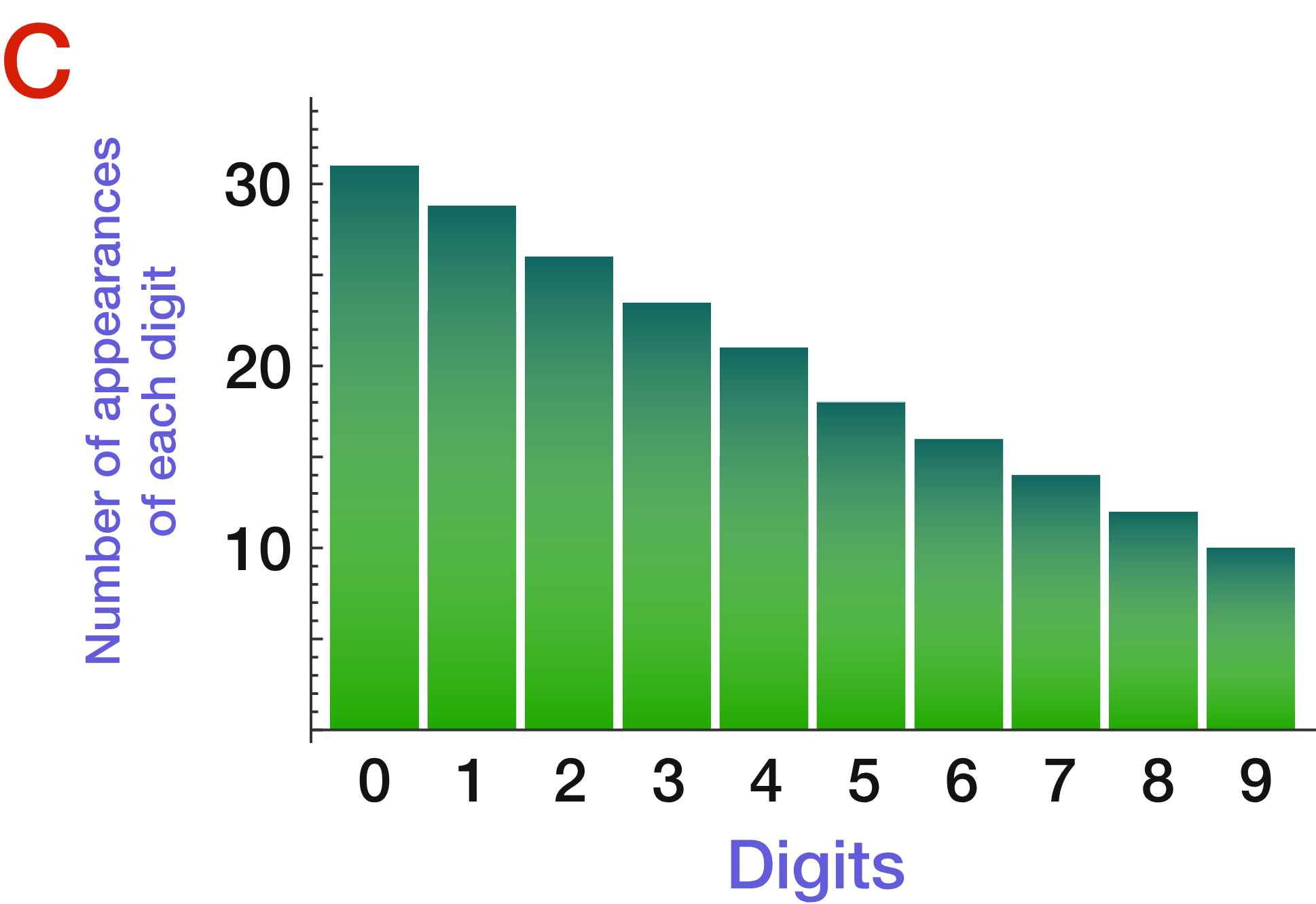digit_string = '12345678901234567890'

for i in range(10):
print('Occurrences of digit', str(i), '->', digit_string.count(str(i)))
Python 3
You need to be connected to run code

# Piercing Lies With Statistics

Suppose, instead of asking people for digits, you use a random number generator to create a string of digits from 0 to 9. Assuming the generator works properly (and every digit is equally likely to occur), which chart do you expect the data to look like now?

Feel free to use the program below to experiment. You can click "Run Code" to generate a list of random digits.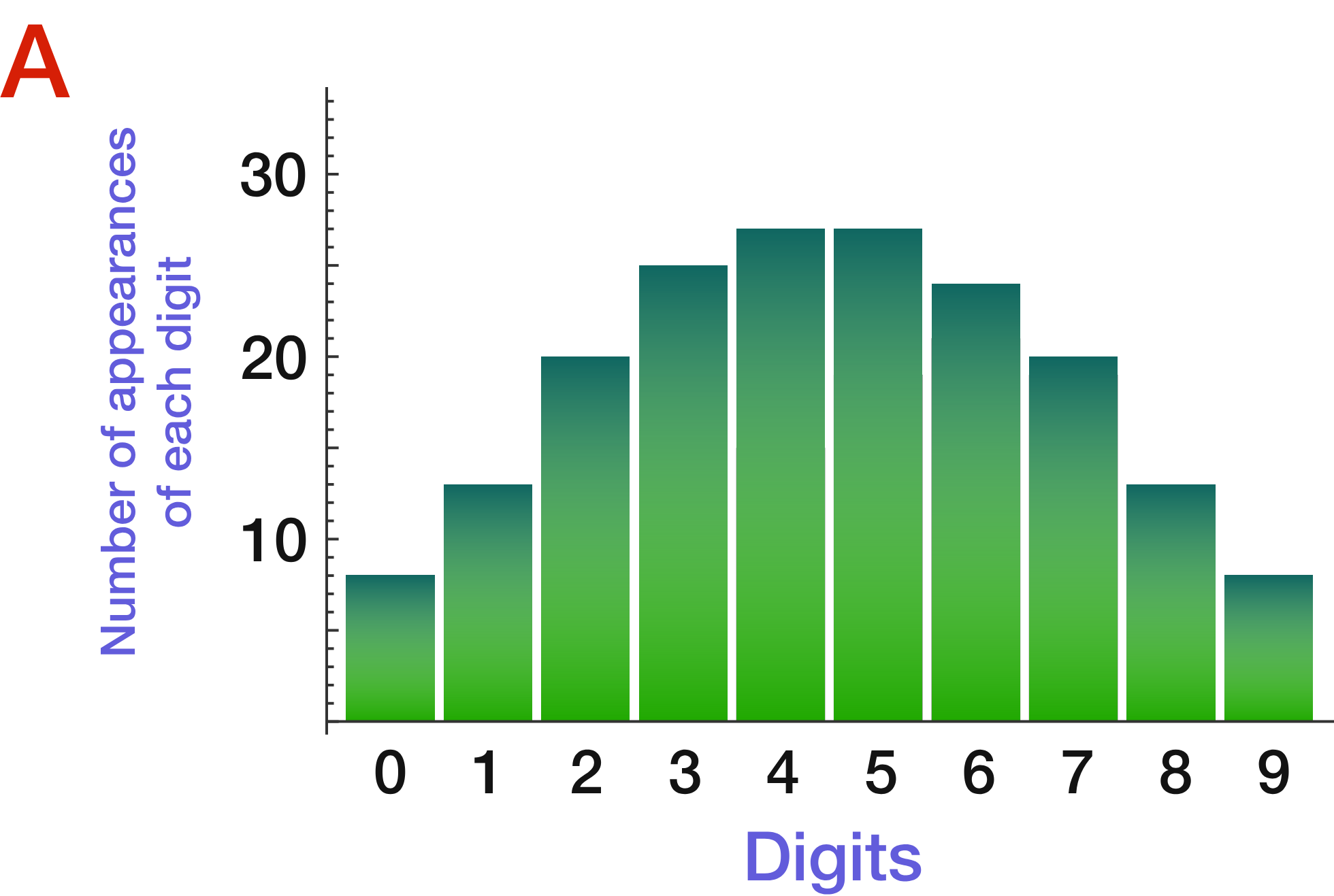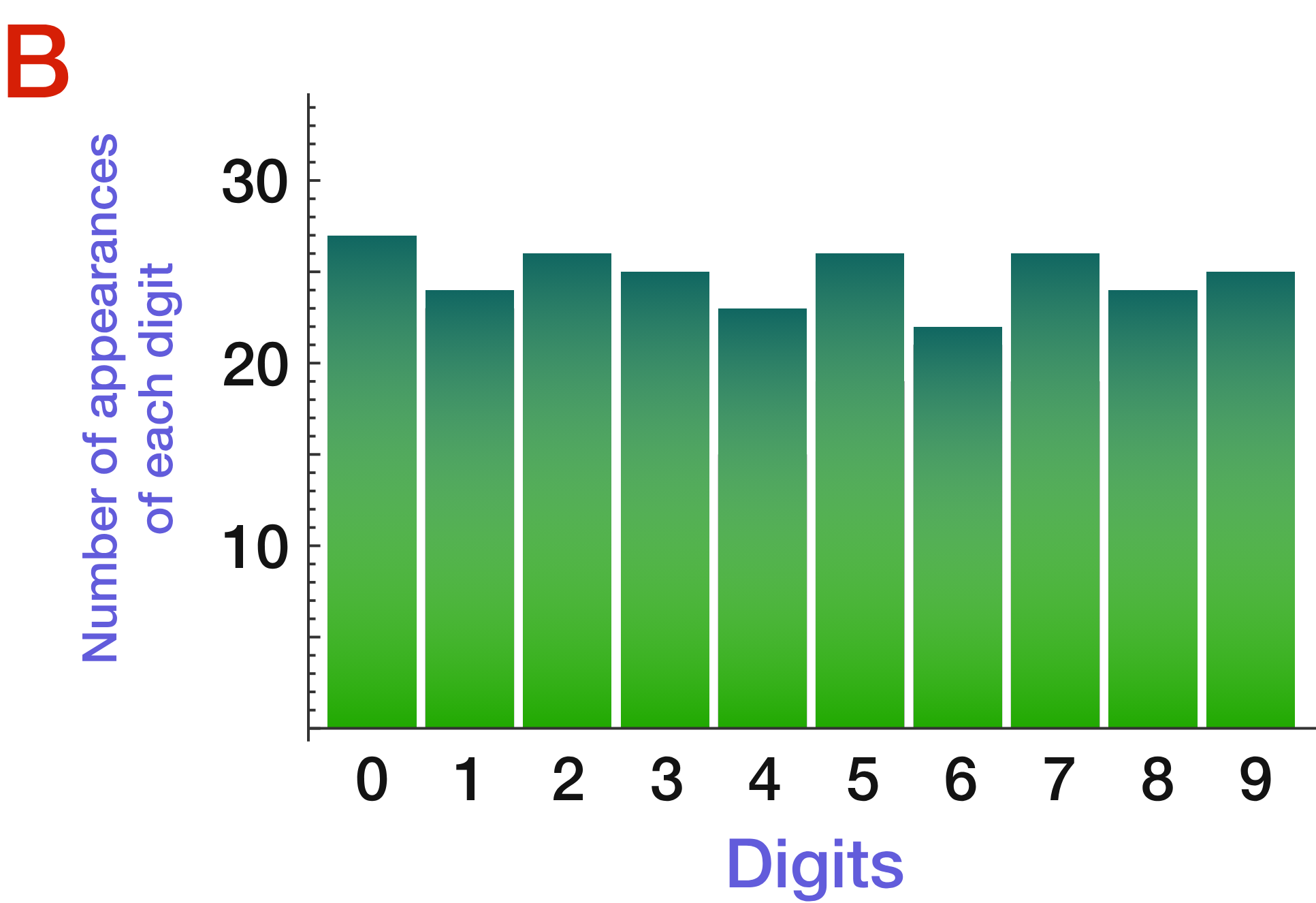import random
digit_string = ''

number_of_random_digits = 80

for i in range(number_of_random_digits):
q = random.randint(0, 9)
print(q, end = '')
digit_string += str(q)

print('')

for i in range(10):
print('Occurrences of digit', i, '->', digit_string.count(str(i)))
Python 3
You need to be connected to run code

# Piercing Lies With Statistics

People attempting to fake numbers without being careful will have various tendencies crop up so that their values won't resemble random data.

This can be used to detect data fakery. If we just look at the last digits of a set of numbers (where rounding or some other bias isn't at play), the digits 0 through 9 should occur with roughly equal frequency.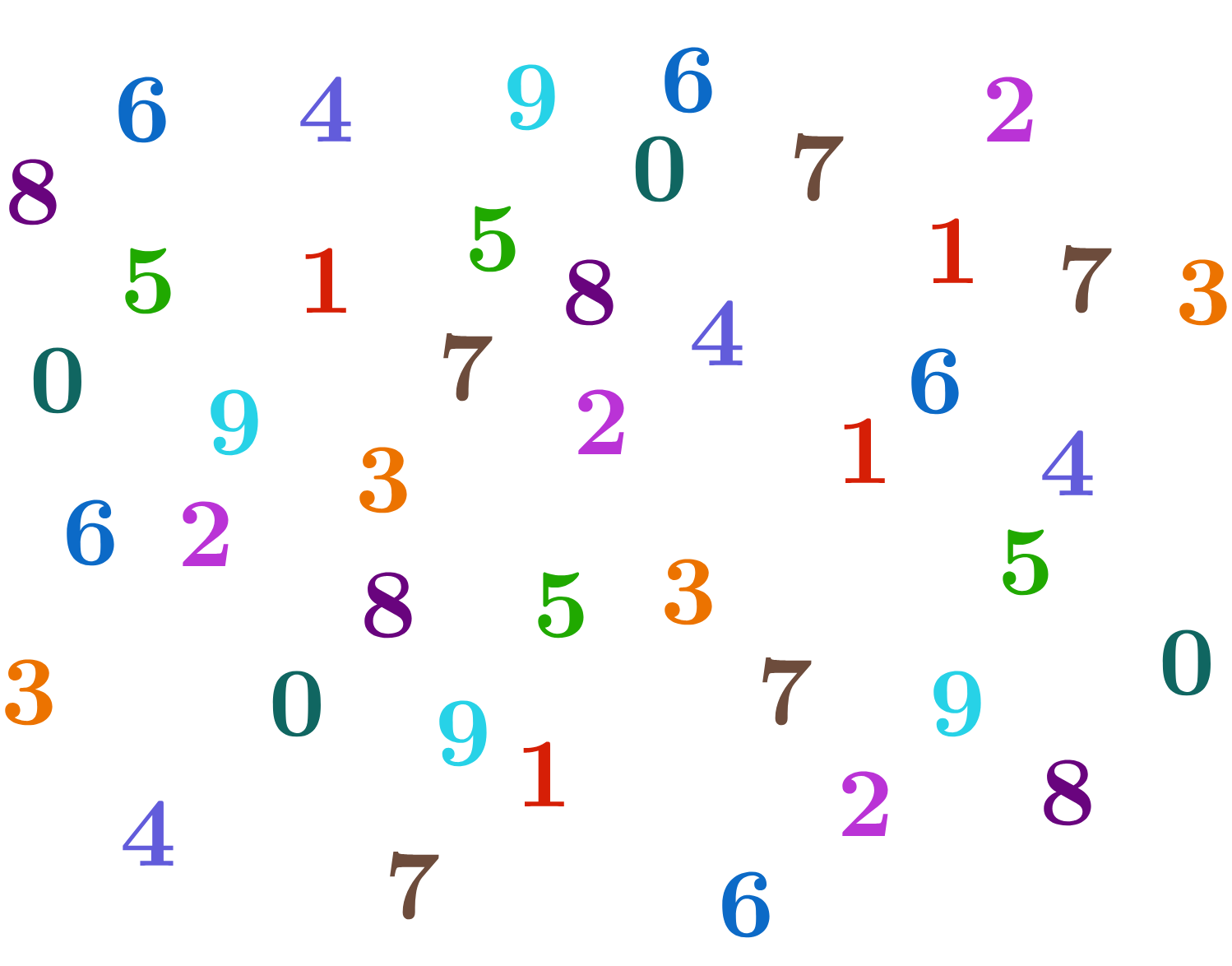# Piercing Lies With Statistics

Is the following financial data of receipts-per-month over a two year period faked?

452.23, 154.23, 152.33, 958.23, 1053.65, 1011.55, 523.19, 319.32, 1234.57, 3923.65, 1132.94, 1231.54, 3832.45, 1231.59, 1209.23, 4918.24, 4324.95, 1103.94, 1342.54, 952.25, 195.24, 923.54, 295.15, 924.24

Base your answer just on the previously mentioned last-digit test. You can assume no biases like specific rounding algorithms. You can also use the "digit counter" program from earlier in this quiz to help.

digit_string = '12345678901234567890'

for i in range(10):
print('Occurrences of digit', str(i), '->', digit_string.count(str(i)))
Python 3
You need to be connected to run code

# Piercing Lies With Statistics

Using the same criterion as before, would the following data be considered faked?

69.1, 62.1, 55.0, 48.9, 46.0, 57.0, 64.9, 66.0, 62.1, 63.0, 59.0, 60.1, 55.0, 59.0, 60.1, 66.9, 72.0, 75.0, 75.9, 87.1, 82.0, 79.0, 75.0, 77.0, 69.1, 59.0, 70.0, 73.0, 68.0, 62.1, 63.0

digit_string = '12345678901234567890'

for i in range(10):
print('Occurrences of digit', str(i), '->', digit_string.count(str(i)))
Python 3
You need to be connected to run code

# Piercing Lies With StatisticsThe previous data was given without context! Let's add some.

The data was from the month of January 1950, at Davis Monthan Air Force Base in Arizona. It represents the highest temperature taken on each day (in Fahrenheit). Since this was 1950, the temperatures were taken and written down by hand.

Given this condition, what is the most likely explanation for the preponderances of "0" digits that end each number?

69.1, 62.1, 55.0, 48.9, 46.0, 57.0, 64.9, 66.0, 62.1, 63.0, 59.0, 60.1, 55.0, 59.0, 60.1, 66.9, 72.0, 75.0, 75.9, 87.1, 82.0, 79.0, 75.0, 77.0, 69.1, 59.0, 70.0, 73.0, 68.0, 62.1, 63.0

# Piercing Lies With Statistics

As the previous question illustrated, remember to ask questions about context. While the fake-detection procedure is used professionally (including by the National Institute of Health) we must always consider the meaning behind a set of numbers; it's possible for data to fail the test but still be real because of some extra consideration (like systematic rounding).

Ask questions like: Do the statistics make sense for the situation? Is there something misleading in the presentation? Is there a bias in how the data is collected? Are categories combined in a deceptive way? Are we assuming anything that isn't true?

Start with the next part of the course, Building Blocks, to begin your journey!

×